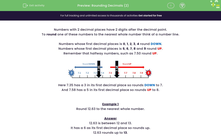# Rounding Decimals (2)

In this worksheet, students must round numbers with two decimal places to the nearest whole number.Key stage:  KS 2

Curriculum topic:   Number: Fractions, Decimals and Percentages

Curriculum subtopic:   Round Up/Down Decimals

Popular topics:   Estimation worksheets

Difficulty level:#### Worksheet Overview

Numbers with 2 decimal places have 2 digits after the decimal point.

To round one of these numbers to the nearest whole number think of a number line.

Numbers whose first decimal places is 01, 2, 3, 4 round DOWN.

Numbers whose first decimal places is 5, 6, 7, 8 and 9 round UP.

Remember that halfway numbers, such as 7.50 round UP.Here 7.35 has a 3 in its first decimal place so rounds DOWN to 7.

And 7.58 has a 5 in its first decimal place so rounds UP to 8.

Example 1

Round 12.63 to the nearest whole number.

12.63 is between 12 and 13.

It has a 6 as its first decimal place so rounds up.

12.63 rounds up to 13.

Example 2

Round 10.38 to the nearest whole number.

10.38 is between 10 and 11.

It has a 3 as its first decimal place so rounds down.

10.38 rounds down to 10.

Example 3

Round 16.50 to the nearest whole number.

16.50 is halfway between 16 and 17 and so we round up.

16.50 rounds up to 17.

### What is EdPlace?

We're your National Curriculum aligned online education content provider helping each child succeed in English, maths and science from year 1 to GCSE. With an EdPlace account you’ll be able to track and measure progress, helping each child achieve their best. We build confidence and attainment by personalising each child’s learning at a level that suits them.

Get started